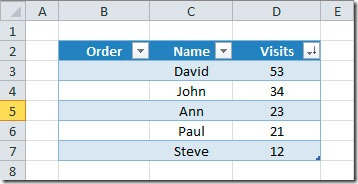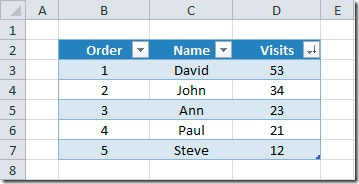### Numbering rows in a Table

When you insert a table on your sheet (by going to Insert-Tables-Table), you may need to number the rows. When you create your table, it will look like this:In this case we want to put and order number on column B. For that we just need to add the following formula into cell B5:

=SUBTOTAL(3,C\$2:C4)

The result will be like this:The formula uses  the SUBTOTAL function with the argument of 3 to COUNTA. This will give the count of all cells in column C, starting to count from the header (C2) and ending on the row that is one cell above the one with the formula. For instance, on cell B5, if you check the formula, it is counting the number of rows from C2 to C4, thus returning the result of 3.

This formula will be updated even if you sort or filter your table data.